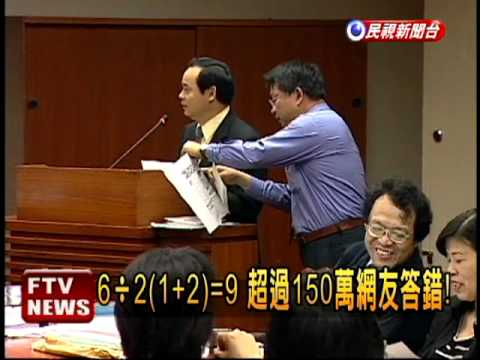# 請問6÷2(1＋2) =?

### 7 個解答

• 最愛解答

致回答者: 神風亨

其實呢條數好多高中生,甚至大學生都計錯

6÷2(3) , 係唔等於 6÷(2*(3))

只有括號入面ge野會係先做 , 其他所有都係由左計到右

所以係計左 6除2先 再乘 3 , 所以答案是9

之所以計錯1 , 我諗係因為中學成日要將出面ge野乘入去

除如 a(b+c) = ab+ac

令人會以為6÷2(3) 要將2乘入去3先 答案 = 1

但如果是這樣 原本 6÷2(1+2) 先乘入去的話會變成 6÷2+4 = 7

6÷2(1+2) 不等於 6÷2(3) ??

所以這種做法是錯的

正確是由左做到右

PS : 如果前面冇野 2(1+2) 同 2(3) 用乘入去ge方法計出黎係一樣的

•登入以回覆解答
• 6÷2(1＋2) 等於?

6÷2(1＋2)

= 6÷2=3

=(1+2)=3

6÷2(1＋2)

=3 3

e.g. "3f" is a Algebra,

"33" =3X3

ANS is "9"

資料來源： me
•登入以回覆解答
• Many people said that the answer is 1. But the actual results should be 9.

Just you know that the steps of a calculation. Then, you will get the result.

First, we calculate the bracket parts.

6÷2(1+2)

=6÷2(3)

Then, we should calculate from left to right.

6÷2(3)

=3(3)

=9

And you get the result of 9.

If you want to know more, please check the following website:&feature=relmfu

資料來源： Myself
•登入以回覆解答
• 想問樓上幾位

如果有一條數: a÷bc 既話 你會點做?

正常既都係 a/(bc)啦

資料來源： 神風亨
•登入以回覆解答
• 你覺得這個解答怎樣？你可以登入投選解答。
• 6÷2(1+2)

=6÷2(3)

=6÷2*3

=9

•登入以回覆解答
• 6÷2(1＋2)

=6÷2(3)

=3(3)

=9

資料來源： 自己
•登入以回覆解答
•登入以回覆解答